Get instant live expert help with Excel or Google Sheets“My Excelchat expert helped me in less than 20 minutes, saving me what would have been 5 hours of work!”

#### Post your problem and you’ll get expert help in seconds.

Your message must be at least 40 characters
Our professional experts are available now. Your privacy is guaranteed.

# How to Check If One Value Exists in a Column

While working on data in Excel, sometimes we need to check whether one value exists in a column or range of data. We tell Excel to find a value in a column or data range, and if the value exists in a column then return a specified value, else return otherwise.

There are a number of ways to accomplish this task, and this article will show you those methods using examples.

## Check if one value exists in a column

When you need to check if one value exists in a column in Excel, you can do this using the MATCH function or VLOOKUP. Here is a description of both with examples.

### Check if a value exists in a column using MATCH

Excel’s MATCH function searches for a value in a column or array and returns its relative position based on your chosen match type, whether exact or partial match. If the value is not found, then it returns a #NA error. Its syntax is:

`MATCH (value, array, [match_type])`

Suppose we have various invoice numbers in a column and their respective amounts. We want to check if a certain invoice exists in that column, and return “YES,” otherwise return #NA. We check this using MATCH and IF functions in Excel, such as

`=IF(MATCH(D3,\$A\$2:\$A\$17,0),"Yes")`

This formula uses the MATCH function as a logical condition and If the MATCH function returns relative position of a value, then the IF function returns “YES,” otherwise this formula returns #NA error as shown below.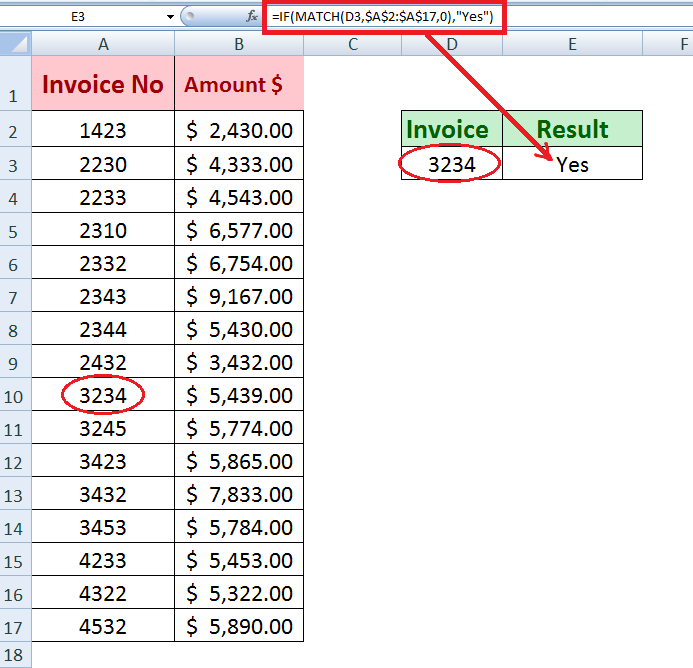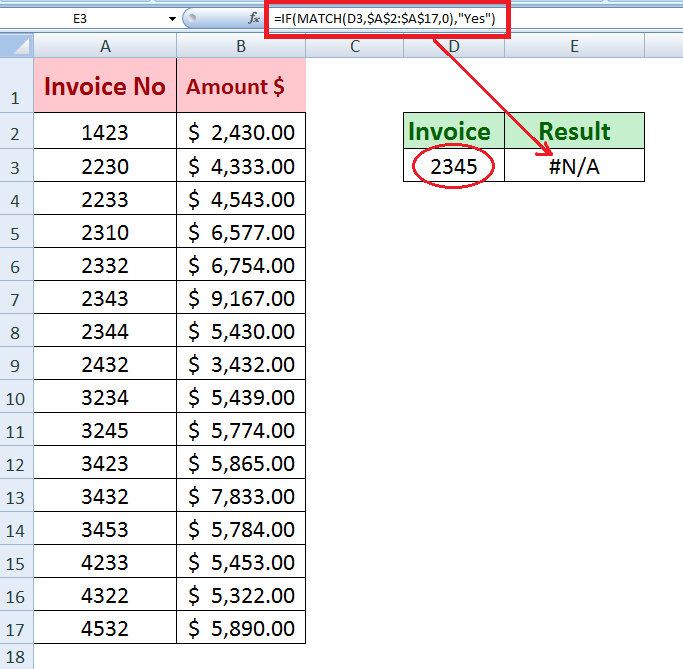If we want to see the result in Yes or No instead of Yes or #NA error, then we need to introduce another function IFERROR in above IF statement formula to return “No” to replace #NA error where value not found.

`=IFERROR(IF(MATCH(D3,\$A\$2:\$A\$17,0),"Yes"),"No")`

The IF function checks whether MATCH function returns a relative position or not? If the MATCH function returns a relative position of a value, then the IF function returns “Yes,” otherwise the IFERROR function replaces #NA error with “No” as shown below.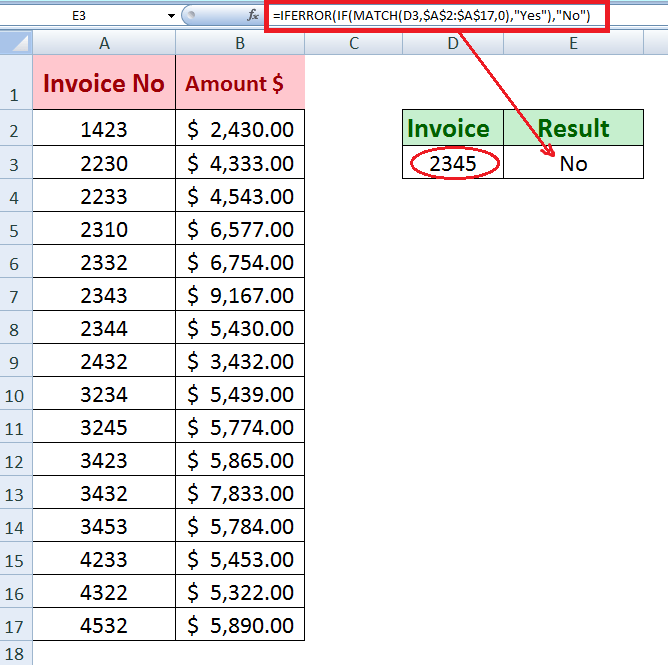### Check if a value exists in a column using VLOOKUP

The VLOOKUP function searches a value in the first column of a table or data range based on match type and returns its related value from that row in a specified column based on column_index_number. IF the value is found in that column then it returns the value as a result. Otherwise, it returns an #NA error.

Its syntax is;

`=VLOOKUP (lookup_value, table_array, column_index_number, [range-lookup])`

Suppose we want to check if a value exists in a column using the VLOOKUP function then return its related value from another column. For example, search an invoice number from the 1st column of a table and return the amount of that invoice. We can do it easily using the VLOOKUP function.

`=VLOOKUP(D3,\$A\$2:\$B\$17,2,FALSE)`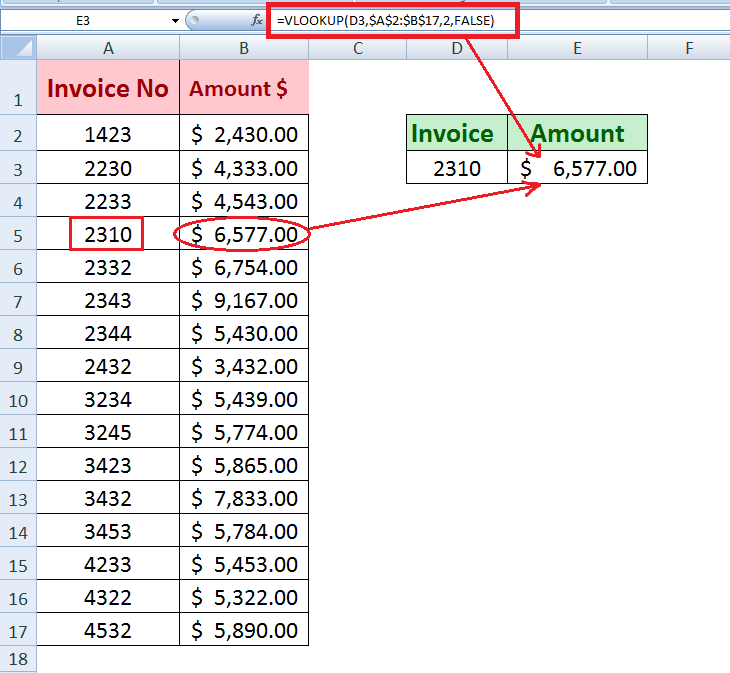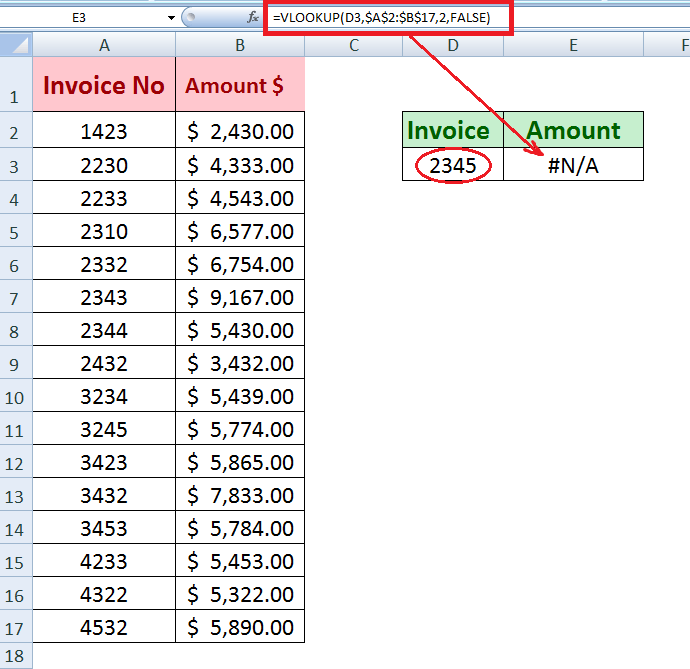Now suppose we want to check if an invoice exists in a column, otherwise return a blank cell. We can do that by introducing the IFERROR function with the VLOOKUP function in a formula to replace an #NA error, such as;

`=IFERROR(VLOOKUP(D3,\$A\$2:\$B\$17,2,FALSE),"")`

We can see this formula returns blank cell when an invoice is not found in the column.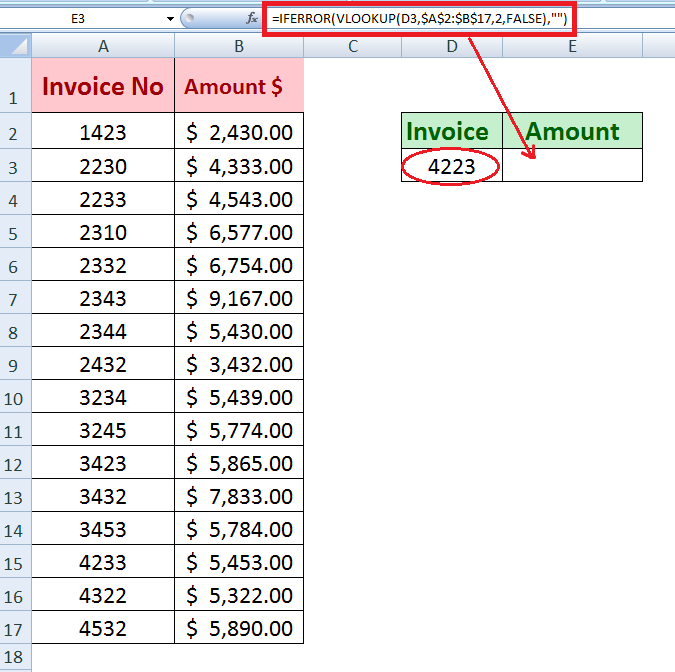In this example, now we need to check if a value exists in a column using VLOOKUP function, and if the value is found then return “Yes”, otherwise return “No”, so we can do this by using the VLOOKUP function with the IF and IFERROR functions, like the following:

`=IFERROR(IF(VLOOKUP(D3,\$A\$2:\$B\$17,2,FALSE),"Yes"),"No")`

In this formula, If the VLOOKUP function searches for an invoice number, then the IF function returns “YES,” otherwise the IFERROR function replaces the #NA error with “No.”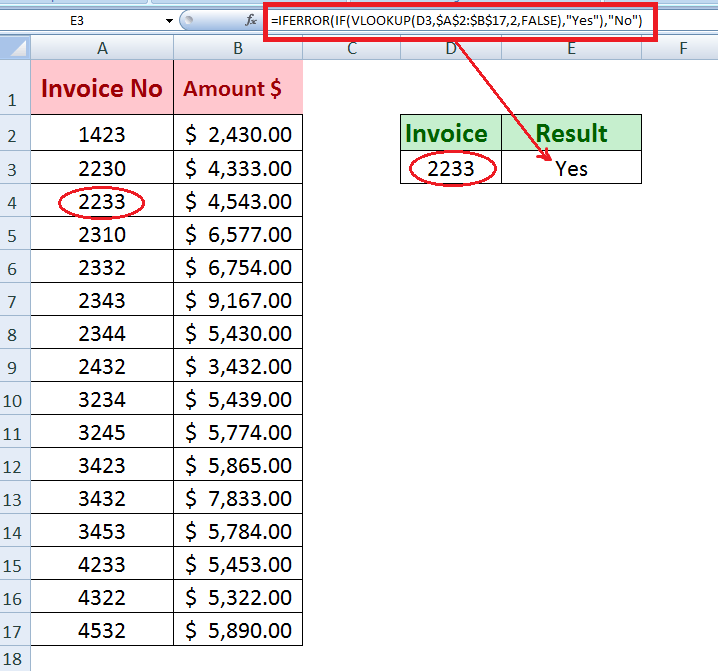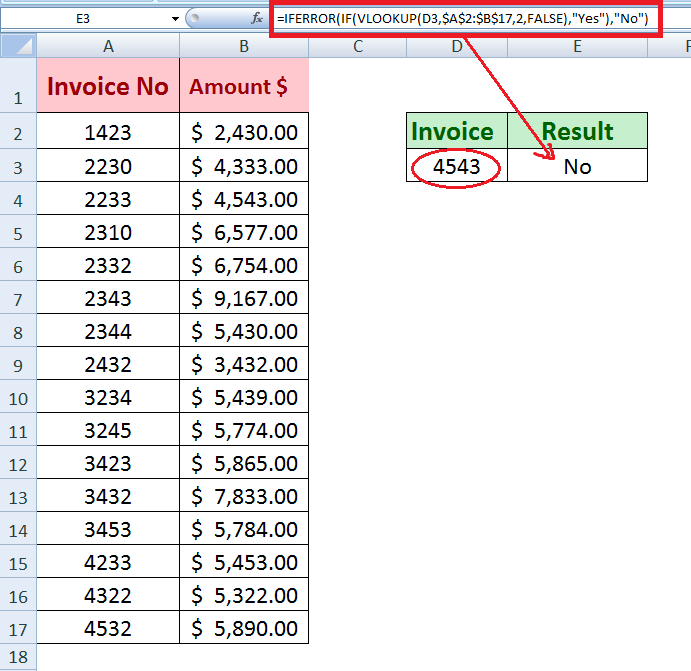### Check if a value exists in a column using Conditional Formatting

The Conditional Formatting feature can be used to check if a value exists in a column or not. If the specified value exists in a column, then conditional formatting highlights that value with an applied formatting style like fill, border, or font, etc.

In this example we want to check if a certain invoice exists in a column using the Conditional Formatting feature and following these steps;

• Select the values in Invoice No. column
• Go to Conditional Formatting > Highlight Cells Rules > Equal to
• A dialog box appears. Insert the value that needs to be searched in column
• Select Formatting Style from the next drop-down list, and press OK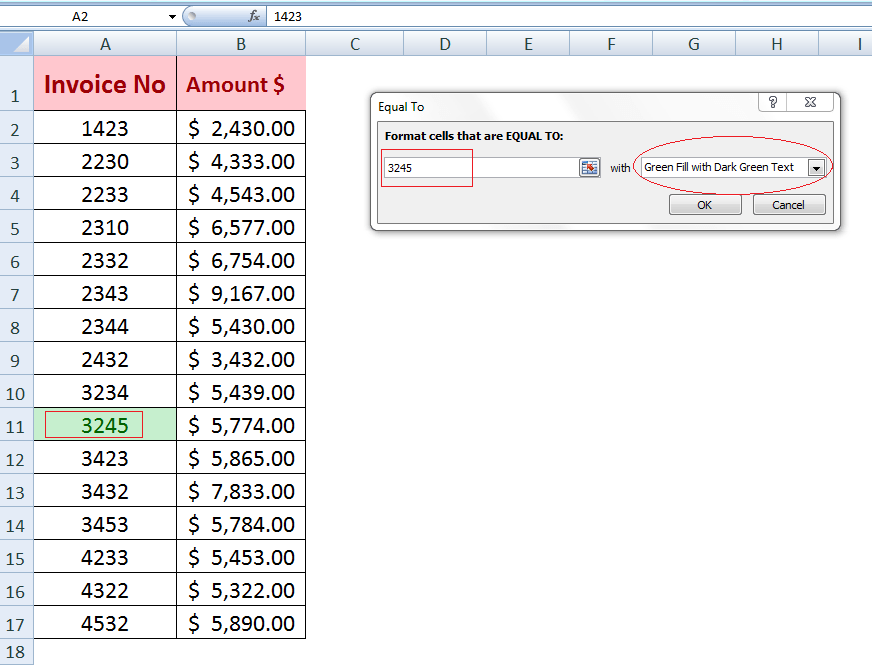The problem you are trying to solve could be more complex than these examples. If you are in a rush or need a real Expert to help you save hours of struggle, click on this link to enter your problem and get connect to a qualified Excel expert in a few seconds. You can share your file, and an expert will create a solution for you on the spot during a 1:1 live chat session. The first session is free.

Related blogs
Solution examplesHow do I make this formula =Value(IFerror(TEXT(AM3,"HMM"),"0")) Return a value of 2400 if the value is not an error?
Solved by F. J. in 21 minsQuestion 5. In cell B18, create a formula using nested IF functions and VLOOKUP functions to determine what to do with the returns. Use the lookup table in the Return Data worksheet. Refer to Figure 8-46 for some hints on how to create the formula.
Solved by O. E. in 33 minsI have a sheet with lots of IFERROR and VLOOKUP formulas. If I want to add two VLOOKUP formulas together, how do I create an IFERROR if no value is returned?
Solved by D. S. in 18 minsI need to use a lookup value that is the result of a formula, (in this case a date) and lookup or match an element in an array (which is a date.. same format as the resultant) and either access the value in the next right cell or use as an index to retrieve it. I have used "=MATCH(C4,Demand,0)" in which C4 is a formula that returns a date in format as an example ... "16-Apr-18" .. and Demand is a series of columns with dates in the same format and sequenced .. I get "#N/A" .. not the value I need and there is an exact date match in the lookup array ....
Solved by V. L. in 23 minsI need help with a nested if statement returning text
Solved by X. B. in 13 mins